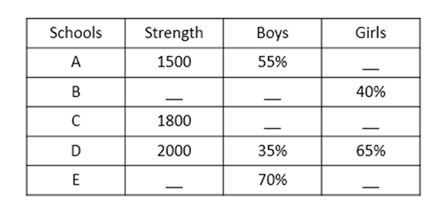# SBI Clerk Prelims 2021 Quantitative Aptitude Questions (Day-67)

Dear Aspirants, Our IBPS Guide team is providing new series of Quantitative Aptitude Questions for SBI Clerk Prelims 2020 so the aspirants can practice it on a daily basis. These questions are framed by our skilled experts after understanding your needs thoroughly. Aspirants can practice these new series questions daily to familiarize with the exact exam pattern and make your preparation effective.

Start Quiz

Table chart

Directions (01-05): Study the following information carefully and answer the questions given below:

The following table represents the strength of five different schools and the percentage of boys and girls in those schools.1) Number of girls in school A is what percent of the number of girls in school D?

A.61.29 %

B.51.92 %

C.55.24 %

D.65.35 %

E.48.78 %

2) If the strength of school B is 2500 and the strength of school E is 3500, number of girls of school E is what percent more than the number of girls of school B?

A.8%

B.6%

C.5%

D.10%

E.None of these

3) If the ratio of the number of boys and number of girls of school C is 5:1 respectively, find the sum number of boys of school C and number of boys of school D.

A.2500

B.1500

C.1000

D.2200

E.None of these

4) If the strength of school B is 2100, find the respective ratio of number of girls of school B and number of girls of school A.

A.56:45

B.45:56

C.46:45

D.45:46

E.None of these

5) Strength of schools A and C together is what percent more than the strength of school D?

A.35%

B.65%

C.55%

D.45%

E.None of these

Missing number series

Directions (6-10): What value should come in the place of (?) in the following number series?

6) 15120, 2160, 360, 72, 18, ?

A.9

B.6

C.18

D.12

E.3

7) 64, 416, ?, 10296, 36036, 90090

A.890

B.996

C.1997

D.1978

E.2288

8) 26, 40, 56, 74, 94, ?

A.106

B.110

C.112

D.116

E.120

9) 3, 28, 197, 986, 2959, ?

A.2960

B.2978

C.3190

D.3280

E.3389

10) 11, 36, 65, 98, ?, 176

A.118

B.125

C.130

D.135

E.120

Percentage of girls in school A = 100 – 55 = 45%

Number of girls in school A = 45/100 x 1500 = 675

Number of girls in school D = 65/100 x 2000 = 1300

Required percentage = 675/1300 x 100 = 51.92%

Percentage of girls of school E = (100 – 70) = 30%

Number of girls of school E = 30/100 x 3500 = 1050

Number of girls of school B = 40/100 x 2500 = 1000

Required percentage = [(1050 – 1000)/1000] x 100 = 50/1000 x 100 = 5%

Number of boys of school C = 5/6 x 1800 = 1500

Number of boys of school D = 35/100 x 2000 = 700

Required sum = 1500 + 700 = 2200

Number of girls of school B = 40/100 x 2100 = 840

Number of girls of school A = (100 – 55)/100 x 1500 = 675

Required ratio = 840 : 675 = 56 : 45

Strength of schools A and C together = 1500 + 1800 = 3300

Required percentage = [(3300 – 2000)/2000] x 100 = 65%

15120/7 = 2160

2160/6 = 360

360/5 = 72

72/4 = 18

18/3 = 6

64 * 6.5 = 416

416 * 5.5 = 2288

2288 * 4.5 = 10296

10296 * 3.5 = 36036

36036 * 2.5 = 90090

42 + 10 = 26

52 + 15 = 40

62 + 20 = 56

72 + 25 = 74

82 + 30 = 94

92 + 35 = 116

3 * 9 + 1 = 28

28 * 7 + 1 = 197

197 * 5 + 1 = 986

986 * 3 + 1 = 2959

2959 * 1 + 1 = 2960

1 * 11 = 11

3 * 12 = 36

5 * 13 = 65

7 * 14 = 98

9 * 15 = 135

11 * 16 = 176

 Check Here to View SBI Clerk Prelims 2021 Quantitative Aptitude Questions Day – 66 Day – 65 Day – 64
5 1 vote
Rating